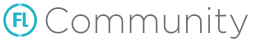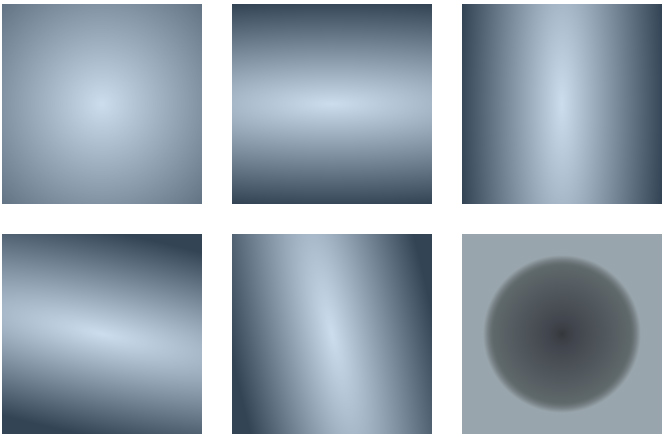I’ve encountered that some SWF assets are losing their’s radial gradients somehow. So I have digged out shapes that exactly have lost their gradients and extracted gradient fill matrix from swflite binary file to the small test project.

``````    package;

import openfl.display.Sprite;
import openfl.display.StageAlign;
import openfl.display.StageScaleMode;
import openfl.geom.Matrix;

class Main extends Sprite {

private static inline var SIZE = 200;
private static inline var GAP = 30;
private var mPos = GAP;

public function new() {
super();

stage.scaleMode = StageScaleMode.NO_SCALE;
stage.align = StageAlign.TOP_LEFT;

// works well in flash, but empty in html5
[3487547, 3882564, 4277581, 6252651, 6713202, 7897735, 9806248, 10069421],
[1, 1, 1, 1, 1, 1, 1, 1],
[0, 17, 44, 222, 229, 240, 253, 255],
[0, -0.0964202880859375, -0.0964202880859375, 0, SIZE * 10, SIZE * 10]);

// works in flash and html5
[6318192, 5857641, 6252399, 6318192],
[0, 1, 0.180392156862745, 0],
[0, 127, 240, 255],
[0.289306640625, 0, 0, -0.289306640625, SIZE * 10, SIZE * 10]);
}

private function testGradient(colors:Array<UInt>, alphas:Array<Float>, ratios:Array<Int>, matrix:Array<Float>) {
var spr = new Sprite();
spr.x = mPos;
spr.y = GAP;

var gfx = spr.graphics;
var mtx = new Matrix(matrix, matrix, matrix, matrix, matrix / 20, matrix / 20);
gfx.drawRect(0, 0, SIZE, SIZE);
mPos += SIZE + GAP;
}
}
``````

So I’ve found gradient matrix with zeroed a and d values that works fine in flash but fails in html5 target. I suppose that this matrix is filled with quite strange gradient setup, but it’s still valid and should work, but it doesn’t.

PS I have reported an issue: https://github.com/openfl/openfl/issues/2404

1 Like

This is a tricky feature to implement

Would be awesome if someone took this sample and tested it against the `CanvasGraphics` code to sort out exactly how this should be implemented!

Let me try to shine a light into this @meps!

gradient is - uhm - quite incomplete - to say the least. The only shape it will ever
produce is a perfect circle, no ellipses ever. Beside that it’s also completely ignoring
the focalPointRatio value.
Luckily there’s hope - it’s a bit tricky though.

The width and height of a gradient are the a and d properties of the matrix fed into the
`createGradientFill()` function.
To retrieve the real width & height in pixels we more or less need to do the reverse of
what the createGradientBox() function does. As you might know it’s supposed to create the
special type of matrix expected by `createGradientFill()`.

To do this, we first need to get the rotation of the gradient represented by the b and a
properties of the matrix. This is done by calculating the arctangent of b & a. Afterwards
we need to divide a or d by the cosine of this arctangent and finally multiply the result
by 1638.4.
The radius of the circle is either the width or height - whichever is the biggest.

Let’s take a look at one of your faulty gradients and it’s matrix:`new Matrix(0.1, 0.5, 0.125, 0, SIZE / 2, SIZE / 2)`

Arctangent = tan^−1(0.5 / 0.1)
Arctangent = 1.373400766945016

Width = (0.1 / cos(1.373400766945016)) * 1638.4
Width = 835.4233571070422

Since the d property of the matrix is 0, the height will also be 0 thus the radius of the
gradient is equal to the width.

Based on the width & height we can then calculate the x & y values we need for the canvas `createRadialGradient()` function. Most of the time those will be zero - unless there’s a different focalPointRatio than 0.
Finally we can use the exact same matrix with the canvas `setTransform()` function. No modifications
needed!

Speaking of zero - you had one interesting edge case:
`new Matrix(0, 0.25, 0.25, 0, SIZE / 2, SIZE / 2)`

This one really took me ages to figure out as a and d of that matrix are both 0 thus no width
or height. Of course my first thought was that the flashplayer uses some default values in that
case. After some more thinking and a lot of trial and error I come to the conclusion it’s as simple
as 1638.4 / 2!

Enough of that dry blabla. I made a fiddle (including a `beginGradientFill()` mockup) with all your faulty radial gradients - working!
https://jsfiddle.net/obscure/fmpwunrc

I’m pretty sure with some slight adjustments this could be used inside CanvasGraphics.hx @singmajesty1 Like

Contributions would be AWESOME

Does `createGradientBox` work properly or is that at fault here as well? That might be an easier place to start in terms of contributing

I’m not sure if I can do this on my own @singmajesty. Though I’m a very good at JavaScript I’m afraid I don’t know enough about OpenFL’s inner workings to fit it in nicely. Did you have a look at the fiddle above? Who worked on CanvasGraphics.hx?

I’ve just looked at at the code. Apparently the internal `createGradientPattern()` function is also used for openfl.display.Graphics.lineGradientStyle which most likely needs to be treated differently.

I’m not sure why your example is so complex. I have added my javascript example that is using exactly the same draw sequence, but with dumb gradient setup with all zeros and only doubled radius as last argument. https://github.com/openfl/openfl/issues/2404#issuecomment-688508891 Can it be that all your calculations are leading to the same values that are close to zero?

So… I have played a little with your code – center.x and center.y are both always zero, and only radius matters. Neither translation tx, ty, nor skew b, c coefficients affect on gradient center coordinates. All of them are applied via matrix transformation.

Now I don’t understand why the radius changes for different gradients. It shouldn’t behave like that in my opinion.

`createGradientBox` works well. Issue is hidden at canvas implementation of flash graphics.

At actionscript you should set type of fill first and then draw as many filled shape as you like.

But at html5 canvas you should draw ‘virtual’ shape first, then set fill type, apply matrix transformation to it and finally call `fill()` method. If you apply transformation matrix to gradient before drawing shape, the shape will be drawn with this transformation too.

So, you should memorize last fill mode, always draw ‘virtual’ shapes and apply fill with transformation only on switching fill type or at the end of shape sequence. I think this will be enough to resolve the problem.

I’m not sure why you’re wondering @meps. Of course it’s more complex!Your sample code just mimics the look of a single gradient using a hardcoded
value for it’s radius. Since we try to replicate the original AS3 `beginGradientFill()`
function we need to calculate the radius dynamically out of the input matrix, as
we need to feed it into the canvas `createRadialGradient()` function.
This is done by these lines:

``````   var angle = Math.atan2(matrix.b, matrix.a);
var cos = Math.cos(angle);
var width = (matrix.a / cos) * 1638.4;
var height = (matrix.d / cos) * 1638.4;

if (width == 0 && height == 0) {
width = height = 819.2;
}

``````

There’s no way to get around.
Furthermore, as I said the `beginGradientFill()` function has an additional parameter:
focalPointRatio

This is taken care of here:

``````   var center = {
x: width * cos * focalPointRatio,
y: height * Math.sin(angle) * focalPointRatio
}
``````

but as I also said the calculated x & y values will be 0 most of the time. It just
differs if the user enters a different focalPointRatio than the default 0 - which
is not the case with your gradients (or more specifically your call to `beginGradientFill()`).
Nevertheless it needs to be calculated that way.

1 Like

I have done fixes to openfl radial gradient graphics, but while testing I’ve encountered some issues.

For example matrices (0.25, 0, 0, 0.25) and (0, 0.25, 0.25, 0) are showing the same radial gradient at flash target, but gradients are differs at html5. I think it’s incorrect to force equal width and height when both a and d coefficients are zeroes.

Another one is wrong focal point behaviour. It looks like html version moves this point farther than flash target does.

Where did you find that gradient conversion formulas?

@singmajesty I have fixed issues: https://github.com/openfl/openfl/pull/2414
Probably needed tests for memory leaks and gradient behavior at edge conditions. But now it works for me as expected.

Oops, looks like I broke other fill types. Will fix it further.

I just merged your pull, it looks like it applies only to the radial type?

If we get this right for canvas we can then copy the same logic for Cairo and probably improve this for native targets

My patch have some issues that I’ve encountered later. I think it’s because of reuse of matrixes pool. Gradients of different movie clip frames are affecting each other depending on their draw order. Needed more investigation to make concrete WTR and fixes, but I have a lack of time at the moment.

And yes it is applied only to radial gradients, linear ones are working right.

At last I have fixed all the gradients issues and brought my fork to some sort of stable state. Now I would like to create a pull request to merge it with the main openfl repository, but don’t know how to merge the current master with my fork to resolve conflicts.

Merged, thank you